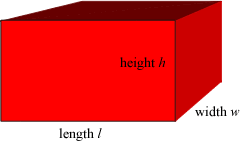index: click on a letter A B C D E F G H I J K L M N O P Q R S T U V W X Y Z A to Z index index: subject areas numbers & symbols sets, logic, proofs geometry algebra trigonometry advanced algebra & pre-calculus calculus advanced topics probability & statistics real world applications multimedia entrieswww.mathwords.com about mathwords website feedback

Rectangular Parallelepiped
Box
Cuboid

A box shape in three dimensional space. Formally, a polyhedron for which all faces are rectangles.

 Rectangular Parallelepiped Volume = lwh Lateral Surface Area = 2lh + 2wh Surface Area = 2lw + 2lh + 2wh